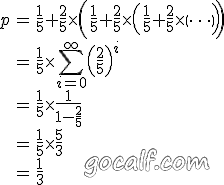======== 分隔符 ========

• 关键词：WordPress，Blog，LaTeX，插件；
• 适用人群：了解 LaTex，需要在 WordPress 搭建的 Blog 内用 LaTeX 书写数学公式的人；
• 平台：WordPress 2.x。

1. 利用 LaTeX 软件或在线服务（如 mimetexLaTeX Equation Editor）生成公式的图片，将图片上传到自己的站点或者 PicasaWeb，然后在文章中插入该图片；
2. 为自己的站点安装 LaTeX 的相关软件，使其可以解析 LaTeX 并生成图片；
3. 利用插件（dahnielson_mimetex），动态地向公开的服务发出请求，获取公式图片并显示。

wordpress.com 的用户都可以在博客中使用一对 $来书写 LaTeX，在 http://support.wordpress.com/latex/ 中有介绍。我的方法就是修改 dahnielson_mimetex，让其调用 wordpress.com 的 LaTeX 服务来生成图片。由于后者比 mimetex 多了一些控制图片颜色和大小的参数，因此要在 dahnielson_mimetex 中做出相应的修改。 首先下载 dahnielson_mimetex v1.2，将其安装到博客上，然后对其进行如下的编辑。 一共只有五处简单的修改，第一处是修改 description，把新加的参数写进去，以免日后忘记： 5,7c5,7 < Description: Use &lt;tex&gt;&lt;/tex&gt; tags to embed LaTeX math in posts, see the <a href="http://www.forkosh.com/mimetex.html">mimeTeX manual</a> for details. < Version: 1.2 < Author: Anders Dahnielson --- > Description: Use &lt;tex bg="000000~ffffff" fg="000000~ffffff" sz="-4~4" escaped="true|false"&gt;&lt;/tex&gt; tags to embed LaTeX math in posts. > Version: 1.2.1 > Author: Anders Dahnielson; Modified by calf (April 12, 2009)  这个插件最初的语法只是 <tex></tex>，我给它添加了四个参数： bg 设定图片的背景色，格式为六个十六进制字符，范围 000000 到 ffffff，默认值为 ffffff 即白色 fg 设定图片的前景色，格式为六个十六进制字符，范围 000000 到 ffffff，默认值为 000000 即黑色 sz 设定图片的大小，格式为整数，范围 -4 到 4，默认值为 0 即正常大小 escaped 设定公式中的 <、>、& 等符号是否是 html 转义的（即用 &lt;、&gt;、&amp; 表示），可取值为 true 或 false，默认值为 false 即未转义的 第二处修改是正则表达式，在 dahnielson_mimetex 类的 parse 函数中，修改过的正则表达式可以匹配刚刚提到的四个参数： 32c32,33 <$regex = '#<tex>(.*?)</tex>#si';
---
> $regex = '#<tex(?:s|bg=["']([w]+)["']|fg=["']([w]+)["']|'. > 'sz=["']([0-9+-]+)["']|escaped=["'](true|false)?["'])*>(.*?)</tex>#si';  第三处修改是提取公式文本和参数，也就是对上面正则表达式的匹配结果做处理： 38,40c39,50 <$formula_text = $match; <$formula_hash = md5($formula_text); <$formula_filename = 'tex_'.$formula_hash.'.gif'; --- >$formula_bg = $match; > if (!$formula_bg) $formula_bg = 'ffffff'; >$formula_fg = $match; > if (!$formula_fg) $formula_fg = '000000'; >$formula_sz = $match; > if (!$formula_sz) $formula_sz = '0'; >$escaped = $match; >$formula_text = $match; > if ($escaped == 'true') $formula_text = htmlspecialchars_decode($formula_text);
> $formula_text_html = htmlspecialchars($formula_text);
> $formula_hash = md5($formula_text.'_'.$formula_bg.'_'.$formula_fg.'_'.$formula_sz.'_1.2.1'); >$formula_filename = 'tex_'.$formula_hash.'.png';  这里我添加了一个变量$formula_text_html，用来记录 html 转义过的公式内容，稍后会看到这样做的目的。

49c59,61
< $mimetex_host = curl_init('http://www.forkosh.dreamhost.com/cgi-bin/mimetexpublic.cgi?formdata='.urlencode($formula_text));
---
> $req_url = 'http://l.wordpress.com/latex.php?latex='.urlencode($formula_text).
> '&bg='.urlencode($formula_bg).'&fg='.urlencode($formula_fg).'&s='.urlencode($formula_sz); >$mimetex_host = curl_init($req_url);  最后一处修改是展示获取到的图片。我给 img 标签加了 class 属性，便于修改样式。添加了 title 属性，当鼠标放在图片上时，可以看到公式内容。注意这里 alt 和 title 都是用 html 转义后的公式内容，这样可以避免公式中的一些特殊字符把 html 结构搞乱： 58c70 < return "<img src="$cache_formula_url" alt="$formula_text" />"; --- > return "<img class="mimetex" src="$cache_formula_url" alt="$formula_text_html" title="$formula_text_html" />";


<tex fg="0000ff" sz="2">\begin{array}{rcl}
p & = & \frac{1}{5}+\frac{2}{5}\times\left(\frac{1}{5}+\frac{2}{5}\times\left(\frac{1}{5}+\frac{2}{5}\times\left(\cdots\right)\right)\right) \\
& = & \frac{1}{5}\times\sum_{i=0}^\infty \left(\frac{2}{5}\right)^i \\
& = & \frac{1}{5}\times\frac{1}{1-\frac{2}{5}} \\
& = & \frac{1}{5}\times\frac{5}{3} \\
& = & \frac{1}{3}
\end{array}</tex>So what do you think? Did I miss something? Is any part unclear? Leave your comments below.